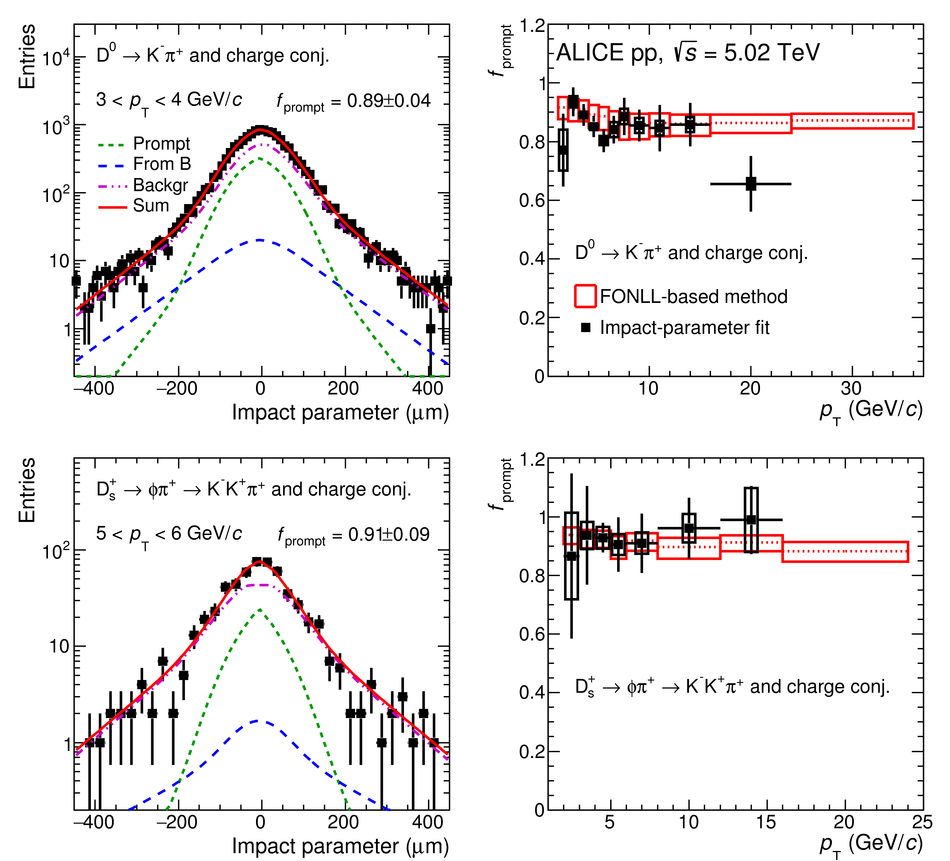# Figure 5

 Left: examples of fits to the impact-parameter distributions of $\Dzero$ and $\Ds$ candidates. The curves show the fit functions describing the prompt, feed-down, and background contributions, as well as their sum, as described in the text Right: fraction of prompt $\Dzero$ and $\Ds$-mesons raw yield as a function of $\pt$ compared to the values obtained with the FONLL-based approach. The results from the data-driven method are shown as square markers with the error bars (boxes) representing the statistical (systematic) uncertainty. The central values of $\fprompt$ from the FONLL-based approach are shown by the dashed line and their uncertainty by the red boxes